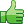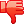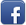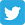# problem with query error

Posted on 16th Feb 2014 07:03 pm by admin

First Thanks to those who helped me on my previous posts, and the following code i'm using is not mine, i grabbed it from here http://www.phpfreaks.com/tutorial/basic-pagination

Now onto the problem...

If i make a search query, i get the following error:

Problem, with Query:Unknown column 'math' in 'where clause'

Not sure were the problem is in the script but here it is

Code: <?php
\$subject = \$_GET['subject'];
\$location = \$_GET['location'];

// find out how many rows are in the table
\$sql = "SELECT COUNT(*) FROM tutorProfiles";
\$result = mysql_query(\$sql) or trigger_error("SQL", E_USER_ERROR);
\$r = mysql_fetch_row(\$result);
\$numrows = \$r;

// number of rows to show per page
\$rowsperpage = 9;
// find out total pages
\$totalpages = ceil(\$numrows / \$rowsperpage);

// get the current page or set a default
if (isset(\$_GET['currentpage']) && is_numeric(\$_GET['currentpage'])) {
// cast var as int
\$currentpage = (int) \$_GET['currentpage'];
} else {
// default page num
\$currentpage = 1;
} // end if

// if current page is greater than total pages...
if (\$currentpage > \$totalpages) {
// set current page to last page
\$currentpage = \$totalpages;
} // end if
// if current page is less than first page...
if (\$currentpage < 1) {
// set current page to first page
\$currentpage = 1;
} // end if

// the offset of the list, based on current page
\$offset = (\$currentpage - 1) * \$rowsperpage;

//retrieve the info from DB
\$sql = 'SELECT * FROM tutorProfiles WHERE subject='.\$subject.' AND location='.\$location.' LIMIT '.\$offset.', '.\$rowsperpage.'';
\$result = mysql_query(\$sql) or die("Problem, with Query:".mysql_error());

// while there are rows to be fetched...
while (\$list = mysql_fetch_assoc(\$result)) {
// echo data
echo '
<div id="profileBox">
<div id="badgeImg">
<img src="images/imgBG.png" name="imgBadge" width="76" height="70" align="left" id="imgBadge" />
<h2>'.\$list['first'].'</h2>
<p>'.\$list['school'].'<br />
'.\$list['degree'] . \$list['class'].'
</p>
</div>
<div id="profleBtn">
<a href="profile.php?id='.\$list['tutorId'].'"><img src="images/linkBtn.png" alt="view Profile" width="150" height="30" border="0" /></a>
</div>
</div>';
} // end while
if(\$result>0){
/*********** Start the pagination links ********/
echo "<p style="clear:both;" align="center">n";
// range of num links to show
\$range = 3;

// if not on page 1, don't show back links
if (\$currentpage > 1) {
// show << link to go back to page 1
echo " <a href='{\$_SERVER['PHP_SELF']}?currentpage=1&subject=\$subject&location=\$location&search2=Search'><<</a> ";
// get previous page num
\$prevpage = \$currentpage - 1;
// show < link to go back to 1 page
echo " <a href='{\$_SERVER['PHP_SELF']}?currentpage=\$prevpage&subject=\$subject&location=\$location&search2=Search'><</a> ";
} // end if

// loop to show links to range of pages around current page
for (\$x = (\$currentpage - \$range); \$x < ((\$currentpage + \$range) + 1); \$x++) {
// if it's a valid page number...
if ((\$x > 0) && (\$x <= \$totalpages)) {
// if we're on current page...
if (\$x == \$currentpage) {
// 'highlight' it but don't make a link
echo " [<b>\$x</b>] ";
// if not current page...
} else {
// make it a link
echo " <a href='{\$_SERVER['PHP_SELF']}?currentpage=\$x&subject=\$subject&location=\$location&search2=Search'>\$x</a> ";
} // end else
} // end if
} // end for

// if not on last page, show forward and last page links
if (\$currentpage != \$totalpages) {
// get next page
\$nextpage = \$currentpage + 1;
// echo forward link for next page
echo " <a href='{\$_SERVER['PHP_SELF']}?currentpage=\$nextpage&subject=\$subject&location=\$location&search2=Search'>></a> ";
// echo forward link for lastpage
echo " <a href='{\$_SERVER['PHP_SELF']}?currentpage=\$totalpages&subject=\$subject&location=\$location&search2=Search'>>></a> ";
} // end if
echo "</p>n";
/****** end build pagination links ******/
}
?>

I hope i'm explaining myself in what my problem is

No comments posted yet

#### Your Answer:

Login to answer
29754##### Other forums

Storing the referrer in session... problems with tabs
Hello All

I am currently working on an ERP project based on the MVC methodology, when a user

Regarding accessing SQL query issued by any user in Oracle 10g
Hi all,
i want to know the queries issued by various users accessing a database...

scandir clients directory
hi,
how can i scandir the clients directory? i need a script that when i click a button it will u

Printing issues with xMII 11.5
For some reason, we have one report (and only one) that is giving us fits when trying to print. The

Table trouble
i have been reading the forum for a few weeks and decided to join. i like the format and the adviceSign up now if you have flare of writing..
Login   |   Register
###### Follow Us